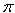SEARCH HOMEMath Central Quandaries & QueriesQuestion from stephanie, a student: The epcot center has a diameter of 265 ft the park needs to paint the park one can will cover 400 sq ft how many cans do they need ?Hi Stephanie.

If you mean the Geosphere, the icon of the Epcot Center park, it is a big sphere that contains the ride Spaceship Earth.

It's not actually a sphere. It's more complicated than that, but since you are given a diameter, I suspect you are expected to treat it as a sphere.

The surface area (this is one side: if you wanted to paint the inside and the outside, you'd have to double this) of a sphere is 4r2, where r is the radius of the sphere. Use this equation to find out how much surface area you have to paint and then divide by the amount of area each can will cover.

The shape of the Geosphere is actually a pentakis dodecahedron (see http://mathworld.wolfram.com/PentakisDodecahedron.html). Furthermore, each of the 60 triangles of the pentakis dodecahedron is further divided into 16 smaller equilateral triangles each of which is a single panel. On each panel, they place four triangular pyramids, making 60 x 16 x 4 x 3 actual triangular surfaces on the exterior of the structure. That's 11 520 sides (ignoring a few that must be removed for the legs and the doors)!

There are lots of interesting facts about it here:
http://www.disneyworldtrivia.com/trivia/epcot/futureworld/spaceshipearth.php

But be warned: the figure they give on that link for the surface area of the sphere is not what you will get using the figure you were given in the question, so don't just copy their answer!

Stephen La Rocque.>Math Central is supported by the University of Regina and The Pacific Institute for the Mathematical Sciences.